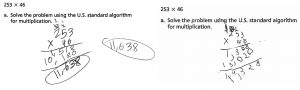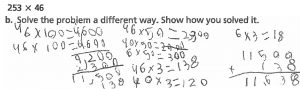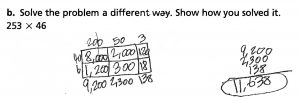Select Page

Last year, my colleague Keith and I worked a few times with a group of 5th grade teachers. One of the questions they asked us to help them think about related to this 5th grade benchmark:

“Fluently solve multidigit multiplication problems using a variety of strategies including the U.S. standard algorithm.”

They told us that they had students who could multiply 2-digits by 2-digits successfully but struggled with 3-digit by 2-digit multiplication problems. They wanted to discuss the complexities in solving 3-digit by 2-digit multiplication problems in general and were also wondering about the use of the U.S. standard algorithm for multiplication with this size numbers.

When solving 3-digit by 2-digit problems, are some methods better than others? Solving problems of this size can be challenging and cumbersome for students. There is so much to keep track of that it is easy to make mistakes, whatever strategy one uses. The U.S. standard algorithm is perhaps the quickest, aside from a calculator. The notation is compact, requiring less recording than other strategies students are likely to develop and use. It involves single-digit multiplication only and doesn’t require paying attention to the magnitude of the sub-products. However, if students are just following the steps of the U.S. standard algorithm, without understanding them, it is easy to lose track of what to multiply, when to add, and what to record where.

Let’s think through some of the issues related to multiplying 3-digit by 2-digit numbers by looking at some 5th grade student work. In an assessment, students were asked to solve 253 x 46 in two different ways, one of which was the U.S. standard algorithm.Kyra and Yannik’s Work

Kyra correctly solved the problem using the U.S. algorithm. It is hard to figure out what Yannik was thinking without asking him, but it is clear that he is not making sense of the steps or the notation for this algorithm.

So how do you help students use the multiplication algorithm with understanding? In Investigations, studying and practicing this algorithm happens at the end of students’ work with multiplication—after they have developed a solid understanding of the operation and “have developed their own fluent methods for solving problems with whole numbers.” (5U4 Teacher Note 3: Studying the U.S. Algorithms) The work connects the U.S. standard algorithm to other strategies, particularly the partial products strategy, and the class talks through the steps and what the numbers represent before students practice the algorithm themselves. However, all this does not ensure that students understand this algorithm. The algorithm was designed to be used without having to really think – it was designed for efficiency – and it masks a lot, particularly the place value of the numerals in the subproducts.

The U.S. standard algorithm is, of course, not the only way to solve 3-digit by 2-digit problems. Interestingly, when asked for a second strategy, Yannik correctly solved it by breaking 253 into 100, 100, 50 and 3 and also breaking 46 into 40 and 6 when needed, in order to multiply 46 by 253:Yannik’s Work

Yes, there are a lot of parts to keep track of. Yes, there are a lot of places to make mistakes. (But Yannik doesn’t.) Yes, it probably took him a while to solve it this way. But Yannik’s thinking is pretty transparent and he seems to understand the mathematics of what he is doing. He breaks the numbers apart in ways that make sense to him and keeps track of what he needs to multiply and what products he needs to add together. He gets the right answer.

For her second way, Kyra broke both factors apart by place and multiplied them. She kept track of the partial products in a rectangle which is similar to the way students use unmarked arrays to solve problems or represent solutions.Kyra’s Work

In both students’ second solution, the magnitude and place value of the factors and products are not hidden, and the partial products are pretty easy to add. It may have taken Kyra a little longer to solve the problem this way compared to using the U.S. standard algorithm, but using the rectangle seemed to make it easy for her to keep track of all the parts.

One question we keep thinking about is: how hard should we push students to use the multiplication algorithm, or any specific algorithm, and for what purpose? Students are encouraged to look for and choose the best strategy for the problem at hand. (How efficient would solving 301 x 50 with the U.S. standard algorithm be?) Choosing the “best strategy” for a problem should also take into consideration what is the best strategy for that particular student to use—one that student can use accurately and efficiently. So, what does that mean for a student who can multiply 3-digit by 2-digit numbers using another strategy besides the U.S. algorithm, but has difficulty with the multiplication algorithm? And what about a student who is struggling to solve 3-digit by 2-digit numbers at all?

As we talked about this with the 5th grade teachers and each other, some more questions bubbled up, related to our longer term goals for students:

• Should students be spending much time solving 3-digit by 2-digit multiplication problems at all without a calculator? What is the purpose?
• How helpful is the multiplication algorithm for students when they move on to multiplying mixed numbers or algebraic expressions?